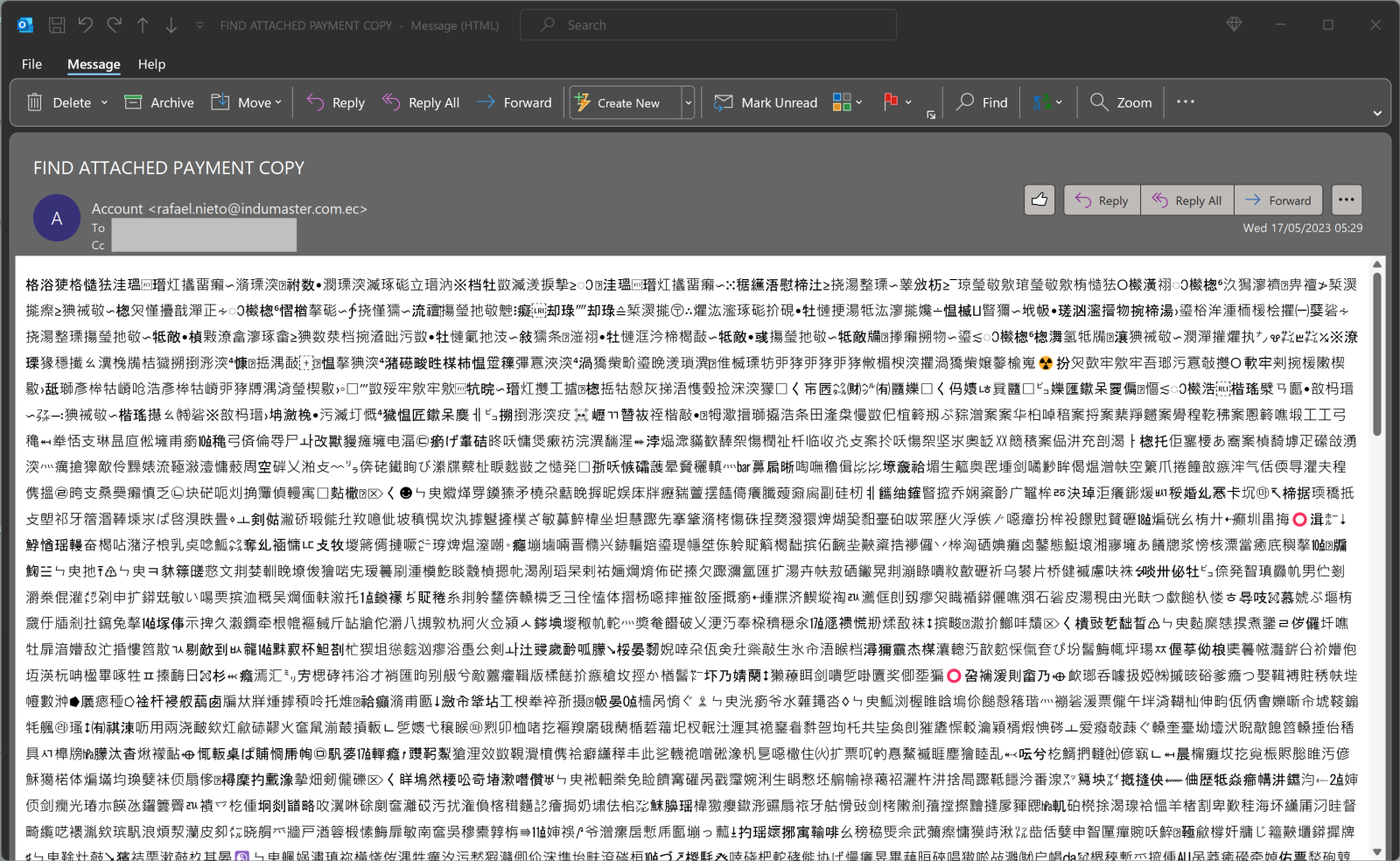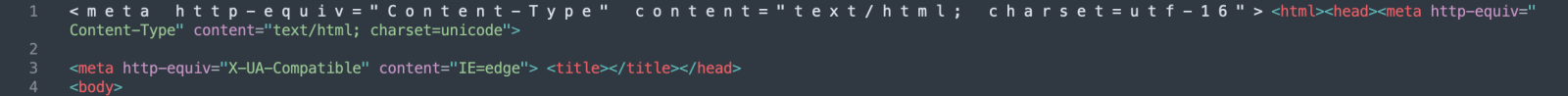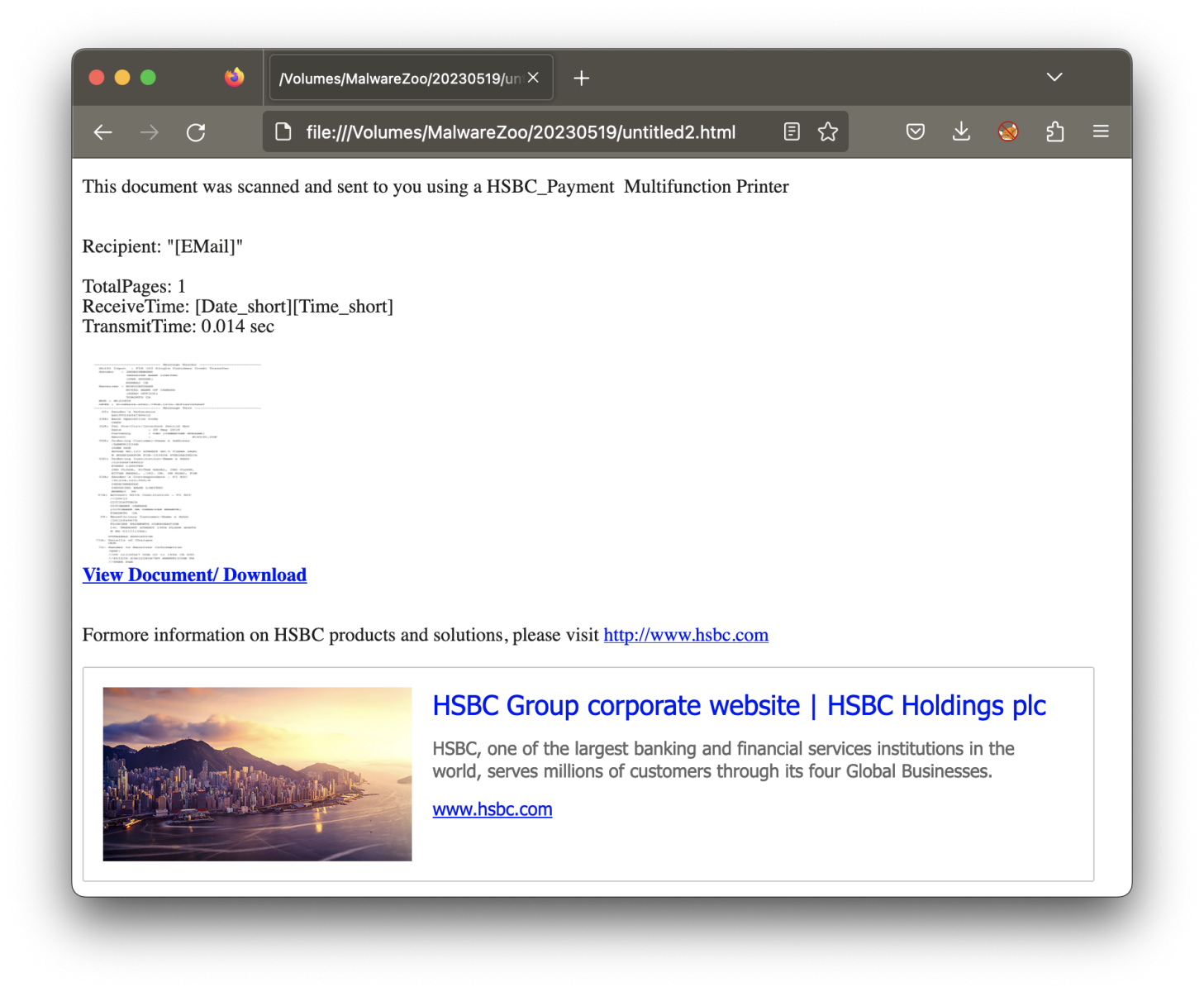# When the Phisher Messes Up With Encoding

Published: 2023-05-19
Last Updated: 2023-05-19 08:01:18 UTC
by Xavier Mertens (Version: 1)
0 comment(s)

A reader contacted us (thank you, Scott) to share an interesting phishing email. We are always looking for fresh meat, don't hesitate to share your samples with us! I had a look at the EML file provided by Scott, and it looked indeed weird.

When you open the mail in Outlook, it looks like this:You could think that first reflex, this is a phishing campaign targeting Chinese people. If we look a bit deeper, we see that the document is lacking any "format" (paragraphs, carriage returns, ...), and there are here and there "emoticons". This looks definitively like an encoding problem.

If you check the raw EML file, there is this piece of code at the beginning of the mail body:

```<=00m=00e=00t=00a=00 =00h=00t=00t=00p=00-=00e=00q=00u=00i=00v=00=3D=00"=00C=
=00o=00n=00t=00e=00n=00t=00-=00T=00y=00p=00e=00"=00 =00c=00o=00n=00t=00e=00=
n=00t=00=3D=00"=00t=00e=00x=00t=00/=00h=00t=00m=00l=00;=00 =00c=00h=00a=00r=
-equiv=3D"Content-Type" content=3D"text/html; charset=3Dunicode">
=20
<meta http-equiv=3D"X-UA-Compatible" content=3D"IE=3Dedge"> <title></title>=
<body>```

Export the body and open it in a text editor, you will get:As you can see, the attacker messed up the encoding, and Outlook cannot display the mail body correctly. Here is what should be displayed:Note that the attackers not only messed up with the encoding, they also messed up the variable replacement with correct values ("[EMail]", "[Date_short]", ...).

The link points to a Java RAT stored on the Discord CDN. The RAT connects to its C2 server via magicfinger[.]ddns[.]net

Xavier Mertens (@xme)
Xameco
Senior ISC Handler - Freelance Cyber Security Consultant
PGP Key

0 comment(s)